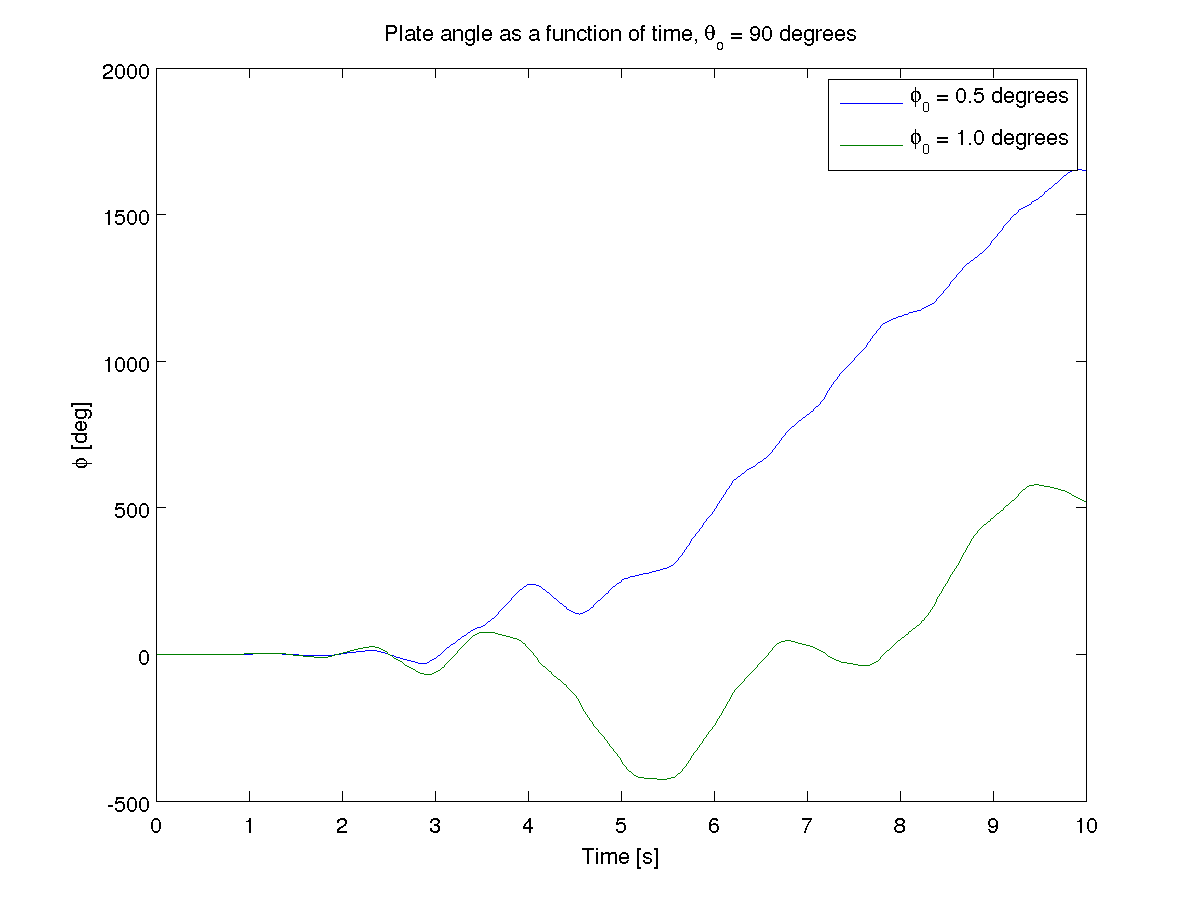# A Chaotic Pendulum Example¶

## System Description¶

This example is a demonstration of a dynamical system that exhibits chaos. It is a simple pendulum with a rotating flat plate in place of the bob. There are two rigid bodies. The first is a uniform rod which rotates by its end about a horizontal axis. The second rigid body is a thin plate which rotates about the rod’s axis. The bodies are suspended in a uniform gravitational field. The rod, $$A$$, has a simple rotation about the Newtonian $$\hat{n}_2$$ axis and the flat plate, $$B$$, rotates about the rod’s $$\hat{a}_3$$ axis. The mass center of the rod is $$l_A$$ from the origin and the flat plate is length, $$l_B$$ from the origin. The plate has mass $$m_B$$, and is symmetric about each axis.

## Generate the equations of motion with sympy¶

#!/usr/bin/env python

# This script generates the equations of motion for a double pendulum where the
# bob rotates about the pendulum rod. It can be shown to be chaotic when
# simulated.

# import sympy and the mechanics module
import sympy as sym
import sympy.physics.mechanics as me

# declare the constants #
# gravity
gravity = sym.symbols('g')
# center of mass length, mass and  moment of inertia of the slender rod
lA, mA, IAxx = sym.symbols('lA mA IAxx')
# center of mass length, mass and moment of inertia of the plate
lB, mB, IBxx, IByy, IBzz = sym.symbols('lB mB IBxx IByy IBzz')

## kinematics ##

# declare the coordinates and speeds and their derivatives #
# theta : angle of the rod
# phi : angle of the plate relative to the rod
# omega : angular speed of the rod
# alpha : angular speed of the plate
theta, phi, omega, alpha = me.dynamicsymbols('theta phi omega alpha')

# reference frames #
# create a Newtonian reference frame
N = me.ReferenceFrame('N')
# create a reference for the rod, A, and the plate, B
A = me.ReferenceFrame('A')
B = me.ReferenceFrame('B')

# orientations #
# the rod rotates with respect to the Newtonian reference frame about the 2
# axis
A.orient(N, 'Axis', [theta, N.y])
# the plate rotates about the rod's primay axis
B.orient(A, 'Axis', [phi, A.z])

# positions #
# origin of the Newtonian reference frame
No = me.Point('No')
# create a point for the mass centers of the two bodies
Ao = me.Point('Ao')
Bo = me.Point('Bo')
# define the positions of the mass centers relative to the Newtonian origin
Ao.set_pos(No, lA * A.z)
Bo.set_pos(No, lB * A.z)

# angular velocities and accelerations #
A.set_ang_vel(N, omega * N.y)
B.set_ang_vel(A, alpha * A.z)

# take the derivative of the angular velocities to get angular accelerations
A.set_ang_acc(N, A.ang_vel_in(N).dt(N))
B.set_ang_acc(N, B.ang_vel_in(N).dt(N))

# linear velocities and accelerations #
No.set_vel(N, 0) # the newtonian origin is fixed
Ao.set_vel(N, omega * lA * A.x)
Ao.a2pt_theory(No, N, A)
Bo.set_vel(N, omega * lB * A.x)
Bo.a2pt_theory(No, N, A)

# kinematical differential equations #
kinDiffs = [omega - thetad, alpha - phid]

## kinetics ##

# rigid bodies #
rod = me.RigidBody('rod', Ao, A, mA, (me.inertia(A, IAxx, IAxx, 0.0), Ao)) # create the empty rod object
plate = me.RigidBody('plate', Bo, B, mB, (me.inertia(B, IBxx, IByy, IBzz), Bo)) # create the empty plate object

## equations of motion with Kane's method ##

# make a list of the bodies
bodyList = [rod, plate]

# forces #
# add the gravitional force to each body
forceList = [(Ao, N.z * gravity * mA),
(Bo, N.z * gravity * mB)]

# create a Kane object with respect to the Newtonian reference frame
kane = me.KanesMethod(N, q_ind=[theta, phi], u_ind=[omega, alpha], kd_eqs=kinDiffs)

# calculate Kane's equations
fr, frstar = kane.kanes_equations(forceList, bodyList)
zero = fr + frstar
# solve Kane's equations for the derivatives of the speeds
# add the kinematical differential equations to get the equations of motion
eom.update(kane.kindiffdict())

# print the results
me.mprint(eom)


## Integration with Matlab¶

The first step is to transfer the equations of motion from SymPy into a function in Matlab.

function xd = state_derivatives(t, x, p)
%function xd = state_derivatives(t, x, p)
% Returns the derivatives of the states as a function of the current state
% and time.
%
% Parameters
% ----------
% t : double
%   Current time.
% x : vector, (4, 1)
%   Current state [theta, phi, omega, alpha].
%
% Returns
% -------
% xd : matrix, 4 x 1
%   The derivative of the current state.

% Unpack the variables so that you can use the sympy equations as is.
theta = x(1);
phi = x(2);
omega = x(3);
alpha = x(4);

% Initialize a vector for the derivatives.
xd = zeros(4, 1);
% Calculate the derivatives of the states. These equations can be copied
% directly from the sympy output but be sure to print with mprint in
% sympy.physics.mechanics to remove the (t) and use Matlab's find and
% replace to change the python ** to the matlab ^. Also note that the
% structure p was used to pass in the parameters and each parameter must
% be prepended with p..

% theta'
xd(1) = omega;
% phi'
xd(2) = alpha;
% omega'
xd(3) = (-2 * p.IBxx * alpha * omega * sin(phi) * cos(phi) + 2 * ...
p.IByy * alpha * omega * sin(phi) * cos(phi) - p.g * p.lA * p.mA * ...
sin(theta) - p.g * p.lB * p.mB * sin(theta)) / (p.IAxx + p.IBxx * ...
sin(phi)^2 + p.IByy * cos(phi)^2 + p.lA^2 * p.mA + p.lB^2 * p.mB);
% alpha'
xd(4) = (p.IBxx - p.IByy) * omega^2 * sin(phi) * cos(phi) / p.IBzz;


## Results from Matlab¶

The pendulum is an interesting system. It is very sensitive to initial conditions. Changing $$\phi$$ slightly when $$\theta$$ is at different angles shows that the system is very unpredictable.